# Series Circuit

(diff) ← Older revision | Latest revision (diff) | Newer revision → (diff)

## Key Stage 3

### Meaning

A Series Circuit is an electrical circuit with only one path for the current to flow.

### About Series Circuits

In a series circuit the current is the same at all locations.
Components placed in series share the potential difference supplied by the power supply.

### Examples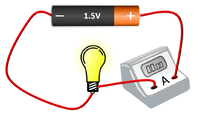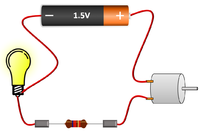The bulb and Ammeter are in series so they have the same Current going through them. The motor and resistor are in series so they have the same Current passing through them but share the 1.5V potential difference between them. The motor, resistor and bulb are in series so they all have the same Current passing through them but share the 1.5V potential difference between them.

## Key Stage 4

### Meaning

A Series Circuit is an electrical circuit with only one path for the current to flow.

### About Series Circuits

In a series circuit the current is the same at all locations.
Components placed in series share the potential difference supplied by the power supply.

### Examples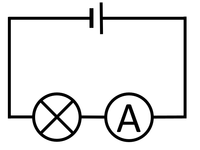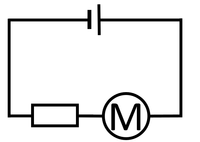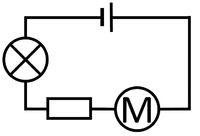The bulb and Ammeter are in series so they have the same Current going through them. The motor and resistor are in series so they have the same Current passing through them but share the potential difference between them. The motor, resistor and bulb are in series so they all have the same Current passing through them but share the potential difference between them.

### Resistors in Series

Adding resistors in series increases the resistance in the circuit.
The resistance of each resistor can be added together.The resistance between points A and B is: 5Ω.The resistance between points A and B is: 2Ω + 5Ω = 7Ω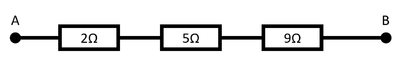The resistance between points A and B is: 2Ω + 5Ω + 9Ω = 16Ω